## Example Questions

### Example Question #21 : Act Math

Suppose the side length of a pentagon is. What is the area of the pentagon?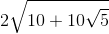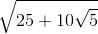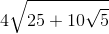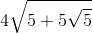Explanation:

Write the formula for the area of a pentagon.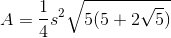Substitute the side length and simplify the radical.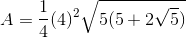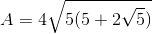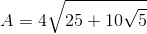### All ACT Math Resources